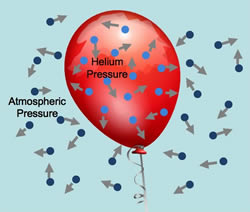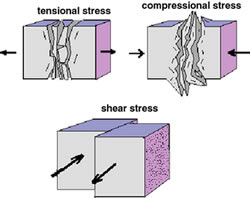# Difference between Pressure and Stress in Physics

##### Key difference: Pressure and Stress are two different types of forces that are constantly working against each other. Pressure is a type of external force that is exerted over a surface area of a material, whereas stress is a type of internal force that acts over a cross sectional area within the material.Pressure and Stress are two different types of forces that are constantly working against each other. Force is an unbalanced force that produces acceleration. There are two main types of forces: internal force and an external force.

Pressure is a type of external force that is exerted over a surface area of a material, whereas stress is a type of internal force that acts over a cross sectional area within the material.Essentially, pressure is the force that is exerted by an object on the area that it impacts, whereas stress is the force that an object pushes back with when it is being deformed, i.e. has force acting on it. For example: Imagine a ball hitting a wall. As the ball hits the wall, it exerts pressure on the wall. Stress the force that the wall exerts back. This is what allows the ball to bounce back.

Comparison between Pressure and Stress in Physics:

 Pressure Stress Definition according to Wikipedia Pressure is the ratio of force to the area over which that force is distributed. Stress is a physical quantity that expresses the internal forces that neighboring particles of a continuous material exert on each other. Definition Pressure is the external force acting over a surface area of a material. Stress is an internal force acting over a cross sectional area within the material. Symbol P or p P or p SI unit Pascal (Pa) Pascal (Pa) Formula P = F / A P = F / A

Image Courtesy: spacegrant.montana.edu, nsb.wikidot.com

Ah jehde reply kr rhe eni english ch :) mera paper e dedo

If Pressure is a external force and since it is only normal to the surface of the body . then what we say if external force is not normal to surface

In equlibrium condition the ext. force acting on the body is equal to the restoring force. In that case you can not simply say that stress acts internally. It can be interchanged too. Not clear.

This ain't shittt

im not satisfied with it..you're just trying to manipulate it..if both of them are just different types of forces..why is pressure a scalar quantity while stress is a vector?!

Actually u r right...but stress is a tensor quantity..not 'vector' n pressure is a special case of tensor having scalar quantity...

thanks for helping us in clarifying our concepts. keep this kind of information which helps students. thanks a lot.